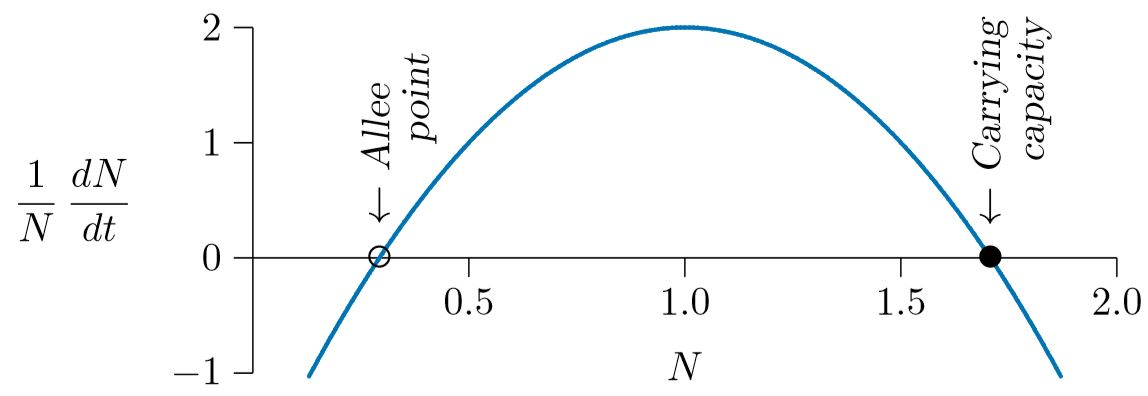# 18.2: One-species Example

$$\newcommand{\vecs}{\overset { \rightharpoonup} {\mathbf{#1}} }$$ $$\newcommand{\vecd}{\overset{-\!-\!\rightharpoonup}{\vphantom{a}\smash {#1}}}$$$$\newcommand{\id}{\mathrm{id}}$$ $$\newcommand{\Span}{\mathrm{span}}$$ $$\newcommand{\kernel}{\mathrm{null}\,}$$ $$\newcommand{\range}{\mathrm{range}\,}$$ $$\newcommand{\RealPart}{\mathrm{Re}}$$ $$\newcommand{\ImaginaryPart}{\mathrm{Im}}$$ $$\newcommand{\Argument}{\mathrm{Arg}}$$ $$\newcommand{\norm}{\| #1 \|}$$ $$\newcommand{\inner}{\langle #1, #2 \rangle}$$ $$\newcommand{\Span}{\mathrm{span}}$$ $$\newcommand{\id}{\mathrm{id}}$$ $$\newcommand{\Span}{\mathrm{span}}$$ $$\newcommand{\kernel}{\mathrm{null}\,}$$ $$\newcommand{\range}{\mathrm{range}\,}$$ $$\newcommand{\RealPart}{\mathrm{Re}}$$ $$\newcommand{\ImaginaryPart}{\mathrm{Im}}$$ $$\newcommand{\Argument}{\mathrm{Arg}}$$ $$\newcommand{\norm}{\| #1 \|}$$ $$\newcommand{\inner}{\langle #1, #2 \rangle}$$ $$\newcommand{\Span}{\mathrm{span}}$$$$\newcommand{\AA}{\unicode[.8,0]{x212B}}$$

To see the basic idea, start with a single species. With variable parameters, population growth for a single species can be written as follows, with the growth rate $$r$$ and the density dependence term $$s$$ depending on the population level $$N$$ and optionally on the time $$t$$.

$\frac{1}{N}\frac{dN}{dt}\,=\,r(N,t)\,+\,s(N,t)N$

Because anything can be embedded in the term $$r(N,t)$$, or in the corresponding $$s(N,t)$$, the equation is perfectly general and can cover any ecological situation for a single species modeled by a differential equation. A basic example, which you have already seen for human population growth, is where the parameters are approximately constant for long periods, but change at certain “bifurcation” events. The parameters for human population growth changed abruptly at the beginning of the modern era, resulting in overall population dynamics that were neither orthologistic nor logistic, but a piecewise combination of the two. In that case the two were blended by varying the parameters as follows.

$$\frac{1}{N}\frac{dN}{dt}\,=\begin{cases}-&0.001185\,&+\,0.00684N,\,&\text{when}\,N\leq\,3.28\,\text{billion}\\&0.03077\,&-\,0.00289N,\,&\text{when}\,N\gt\,3.28\,\text{billion}\end{cases}$$

This led to the population growth curve of Figure 6.3, which nicely modeled human population growth over the centuries.

Human population growth called for a piecewise blending of the parameters, because the parameters changed rather abruptly from one constant set to another. The parameters can also be blended continuously for parameters that change gradually.

For example, take an orthologistic equation, $$1/N\,dN/dt\,=\,−2+2N$$, and a logistic equation, $$1/N\,dN/dt\,=\,4−2N$$, and consider $$N$$ as it ranges from 0 to 1. Have the orthologistic equation apply exactly as $$N$$ approaches 0, and the logistic equation apply exactly when N reaches 1. Then let $$r$$ change uniformly from −2 to +4 and $$s$$ change uniformly from +2 to −2 as $$N$$ goes from 0 to 1, as follows.

$$r(N,t)\,=6N-2\\s(N,t)\,=-4N+2$$

Plugging that into Equation 18.1 gives

\begin{align}\frac{1}{N}\frac{dN}{dt}&=\,(6N-2)\,+\,(-4N+2)N\\&\,=\,-2\,+\,8N\,-4N^2\\&\,=\,r\,+\,sN\,=s_2N^2\end{align}

This kind of blending between orthologistic and logistic has simply added one more term to the population growth equation, an $$N^2$$ term—one of the terms proposed by Hutchinson (Equation 4.2). The result is graphed in Figure $$\PageIndex{1}$$.Figure $$\PageIndex{1}$$. Orthologistic and logistic equations smoothly blended.

This smoothly blends the orthologistic, which has an Allee point but no carrying capacity, with the logistic, which has a carrying capacity with no Allee point, providing both in the blended curve of Figure $$\PageIndex{1}$$. The curve has an Allee point at about $$N\,=\,0.3$$ and a carrying capacity at about $$N\,=\,1.7$$. Compare this with the piecewise blending depicted earlier in Figure 4.4.

This page titled 18.2: One-species Example is shared under a CC BY-NC 4.0 license and was authored, remixed, and/or curated by Clarence Lehman, Shelby Loberg, & Adam Clark (University of Minnesota Libraries Publishing) via source content that was edited to the style and standards of the LibreTexts platform; a detailed edit history is available upon request.